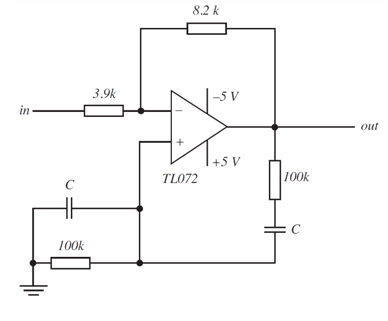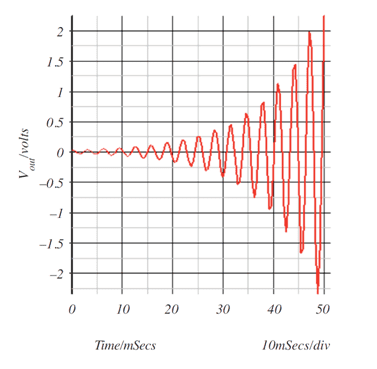# Wien Bridge Help

## Homework Statement

When testing the circuit of FIGURE 1 (a) it was found that the output shown in FIGURE 1 (b) was obtained when the input was connected to ground.explain why this was so and attempt an estimate of the capacitance values of C.

## The Attempt at a Solution

estimating the waveform as 7ms the formula f = 1/T was used to get the frequency of 142.857Hz

assuming C1 = C2 and R1 = R2

the formula f = 1/2πCR was used, rearranged into C= 1/2πfR to give capacitance as 111uF.

any help is much appreciated in giving me any advice as to whather this is correct.

#### Attachments

Last edited by a moderator:

Merlin3189
Homework Helper
Gold Member
estimating the waveform as 7ms the formula f = 1/T was used to get the frequency of 142.857Hz
I don't see T = 7 ms
I count about 10 peaks in the last 30 ms , or about 3&a bit cycles between 20 and 30 ms.
assuming C1 = C2 and R1 = R2
I see no R!, R2, C1, C2
The two R values are given and the two capacitors are marked the same, C , so no assumptions needed.
the formula f = 1/2πCR was used, rearranged into C= 1/2πfR to give capacitance as 111uF.
.
Ok formula, but I don't get 111 uF, with either your frequency or mine.

The shown circit is a so called "WIEN bridge oscillator".
And oscillator has no signal input at all - hence, it is quite logical that the 3.9k resistor must be connected to ground.

Merlin3189
Homework Helper
Gold Member
The shown circit is a so called "WIEN bridge oscillator". ...
... the 3.9k resistor must be connected to ground.
.
Do you not think the question wanted an explanation of the WBO?
I thought they did not sayWBO so that the reader would tackle it as a simple op-amp circuit with a Wien Bridge feedback circuit, explain the effect and magnitude of the WB fb, the choice of resistors for the negative fb and the significance for the form of the graph.

I expect you are supposed to recognise it as a Wien Bridge circuit, else some of us would struggle to calculate the properties of the +ve fb cct from scratch.

And just out of interest, would the circuit not oscillate if the input were at some other potential? (such as +1.5 V or - 0.7 V, not +13.8V etc.)

Do you not think the question wanted an explanation of the WBO?
In the problem formulation I did not read the term "oscillator". So I was not sure if the questioner did know that the circuit will produce self-contained oscillations.
More than that, from the first sentence Ive got he impression that he was surprised to observe any output without an input signal.
That was he background of my answer.
It was not my intention to explain the oscillator principle - including loop gain, oscillation condition, start conditions, amplitude control ......

PS: Why didnt YOU try an "explanation of the WBO"?

Merlin3189
Homework Helper
Gold Member
... PS: Why didnt YOU try an "explanation of the WBO"?
I'm sure you know the answer to that! But, nice way to sidestep the question about having a non-zero input.

And of course I'm not allowed to say that, as it is a HW Q.

As you say, whatever the intention of the Q, recognising the WB cct is the key.

When I first saw it, I thought that it was an unstable amplifier. The output shows it bursting into oscillation (as one I might build possibly would!).
It's the " ... explain why this was so ..." that made me think that they were looking for more than "it's a WBO." But perhaps not. If youhave a name or a formula, that's all you need these days. Wolfram will do the rest.

But, nice way to sidestep the question about having a non-zero input.
Was this a serious question? Both of us know how a dc vltage can shift the operational point...

If you have a name or a formula, that's all you need these days. Wolfram will do the rest.
No, I dont think so.
Oscillators are, in particular, sometimes tricky circuits.
I am sure, Wolfram does not know the difference between a necessary and a sufficient oscillation condition.
There are, for example, some circuits with feedback showing oscillatory behaviour in simulation packages but not in reality.

(Comment: Do you - as a "retired teacher" - really recommend to use Wolfram for beginners who should gain an understanding of the working principle of electronic circuits?)

Last edited:
Merlin3189
Homework Helper
Gold Member
No, it wasn't a serious question. Just referring to your first comment
... the 3.9k resistor must be connected to ground.
But I shouldn't tease,sorry.

Turns out you're right about Wolfram. It offered me a choice of bridges in Vienna. I'm very disappointed.

While I would not want a student of mine to work that way, it seems to me the current general view is that no one needs to understand anything, as computers can do it for you. I thought Stephen Wolfram was further on the way to making this a reality. I'm confident they're already a lot smarter than me at any rate!

While I would not want a student of mine to work that way, it seems to me the current general view is that no one needs to understand anything, as computers can do it for you.

To me, this sounds a bit pessimstic (sarcastic?)